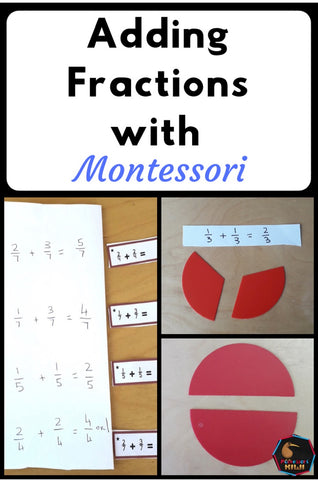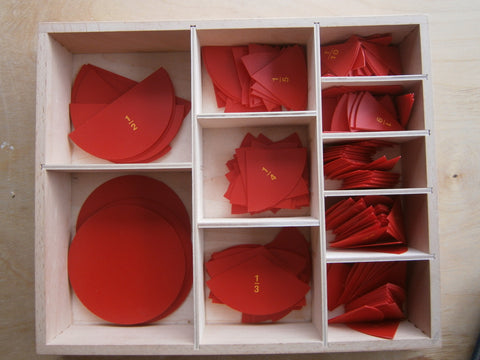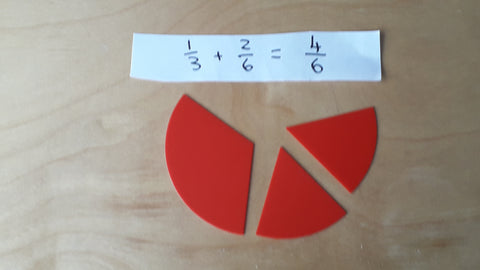Adding Fractions with Montessori equipment helps students visualise what parts of a whole look like. Using hands on materials the child is able to make a visual imprint of what certain fractions look like

The lesson - SAME DENOMINATORS

Prior to this lesson students will need to

• have been introduced to different fractions and practised drawing them into booklets for example drawing 1/6 or 1/4

2) Have the montessori fraction pieces (the box of different pieces works best)3) Take out a large fraction such as a third. Ask student what it is. Do you know how many of these make a whole? If they don't tell the student the answer

4) Today we are going to do an equation with thirds but it won't quite equal a whole

5) Write 1/3 + 1/3 on a piece of paper. Place 1/3 on the table and then get out another third. Say what you are doing I had 1/3 now I am adding another third.

6)How many thirds do I have now 2/34) repeat with another fraction e.g. 2/4 + 1/4.

5) Students then practise different equations with the addition of fraction tickets

--------------------

The lesson - DIFFERENT DENOMINATORS - one change

Prior to this lesson students will need to:

• Be very fluent in adding fractions with the same denominators
• Be fluent with equivalent fractions click the link to see my lesson on equivalent fractions.

1) Get out 1/3 and two sixth pieces.

3) If needed prompt about equivalent fractions are there any pieces here we could change to being something else?

4) Let's change the third. It is the same as 2/6

5) We could rewrite this as 2/6 + 2/6

6) That is 4/6

7) Repeat with another equation this time bring to the students attention that you are changing the larger fraction (the one with the smaller denominator) into smaller pieces.

8) Use the fraction addition tickets for independent practiseThe lesson  -DIFFERENT DENOMINATORS - two changes

1) Take out 1/2 and 1/5 Have the student try and add them together using the equipment to help them

2) Stop and look at the written equation. Say to the students I can see this one has a denominator of 2 and this one has a denominator of 5. I know that 2 and 5 are both in the 10x table. I think if I can get both of these denominators to be 10 I could add them together

3) Write 1/5 = _/10  Say I have multiplied the bottom number by two. I will do the same to the top to make it fair this means I have 2/10

4) I will do the same with the 1/2. Write 1/2 = _/10. Say I have multiplied the bottom number by five. I will do the same to the top to make it fair. This means I have 5/10.

6) I have 7/10

7) Practise with the fraction tickets.

Interested in More Montessori Curriculum resources? Find them here

You can join my mailing list to get more montessori resources, notifications of new products and news here